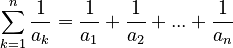# Problems Involving Sequences

(diff) ← Older revision | Latest revision (diff) | Newer revision → (diff)

# Problems with Sequences

This is a small list of exercises involving sequences of numbers, for beginners. They do not require storage, thus they have solutions without using arrays.

## Problems with sequences (no arrays needed)

### Even Elements

How many even elements are in a sequence of n integer numbers?

### Signed Elements

How many negative, zero, and positive elements does a sequence of n numbers contain?

### Sum and Product

Compute the sum and the product of numbers 1 to n, n given. Is there a complexity difference between the best algorithms you can find for the two?

### Find Element

Given a number a and a sequence of n elements, on what position does a appear in the sequence?

### Indices and Elements

How many numbers in a sequence of n elements are equal to the position in which they appear in the sequence?

### Increasing Sequence

Are the numbers in a sequence in increasing order?

### Maximum and minimum

Compute the maximum and the minimum value in a sequence of n numbers.

### Fibonacci numbers

Compute the nth number in the Fibonacci sequence. The Fibonacci sequence has f1 = 0, f2 = 1 and fn = fn-1 + fn-2. Example: 0 1 1 2 3 5 8 13 21 ...

### Monotonic Sequence

Is a sequence of numbers monotonic? We define a monotonic sequence as a sequence whose numbers are either in increasing order, or in decreasing order.

### Identical Numbers

What is the maximum number of consecutive identical numbers in a sequence of n elements?

Given a sequence of numbers a1 a2 ... an compute the summation$\sum _{{k=1}}^{n}{\frac {1}{a_{k}}}={\frac {1}{a_{1}}}+{\frac {1}{a_{2}}}+...+{\frac {1}{a_{n}}}$

### Word Counting

How many groups of consecutive non-zero numbers are in a sequence? Consider such groups as words, zero being a divider such as space.

### Rotating Sequence h

Let's define a rotating increasing sequence as a sequence of numbers that is either in increasing order, or can be transformed into one by successive rotations (the last element becomes first). Problem: is a sequence of n numbers a rotating increasing sequence?

### Rotating Monotonic Sequence h

Same as before we define a rotating monotonic sequence as a sequence that is either monotonic, or can be made into one by rotations. Problem: is a sequence of n numbers a rotating monotonic sequence?

### Bitonic Sequence h

Is a sequence of numbers bitonic? We define a bitonic sequence as a sequence whose numbers are begin in increasing order, and then start decreasing and remain decreasing until the end of the sequence. Increasing and decreasing sequences are also bitonic sequences (that is, it's allowed to skip one of the two sections).

### Rotating Bitonic Sequence h

Same as before we define a rotating bitonic sequence as a sequence that is either bitonic, or can be made into one by rotations. Problem: is a sequence of n numbers a rotating bitonic sequence?

### Brackets h

Given a sequence of 0 and 1, where 0 means open bracket and 1 means close bracket, find out if the sequence represents a correct sequence of brackets, and if so, compute the maximum number of nested brackets. Example: 0 1 0 0 1 0 1 1 is correct and has a maximum nesting factor of 2, while 0 0 1 1 1 0 is incorrect.

Legend:

• h means hard. The problem is difficult.